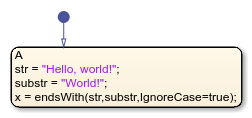# endsWith

Determine if strings end with pattern

## Syntax

``tf = endsWith(str,pat)``
``tf = endsWith(str,pat,IgnoreCase=true)``

## Description

example

````tf = endsWith(str,pat)` returns `1` (`true`) if `str` ends with the specified pattern, and returns `0` (`false`) otherwise.```

example

````tf = endsWith(str,pat,IgnoreCase=true)` ignores case when determining if `str` ends with `pat`.```

Note

Only Stateflow® charts that use MATLAB® as the action language support the `startsWith` operator.

## Examples

expand all

Return `1(true)` when the string ends with the specified pattern.

```h = "Hello, world!" pat = "world!" x = endsWith(h,pat)```Return `1(true)` when the string ends with the specified pattern, regardless of case.

```h = "Hello, world!" pat = "World!" x = endsWith(h,pat,IgnoreCase=true)```## Version History

Introduced in R2021b